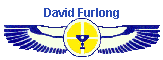Next Trip December 2013               Next Trip December 2013               Next Trip December 2013Information Itinerary Abydos Information Accommodation Booking Contact Egypt Home Page Site Home Page Articles Hatshepsut's Mortuary Temple - Solstice alignments. Sekeds and the Geometry of the Egyptian Pyramids. Sekeds and the Geometry of the Great Pyramid The Osirion and the Flower of Life The Astronomical Alignment of Egyptian Temples. Blog Diary 2007

Mid-Winter Solstice Celebration Holiday 2013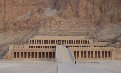A fabulous week holiday in Luxor visiting many ancient sites culminating in celebrating the mid-winter solstice at the magnificent temple of Queen Hatshepsut.

16th - 23rd December:

An excellent book by Richard Wilkinson describing the different temple sites of Ancient Egypt.

A clear concise book on the history of Egypt.

The definitive book on the pyramids.

Information on all of the gods and goddesses of Ancient Egypt.

An excellent guide to learning hieroglyphs.

Detailed information on one of the most famous sites in Egypt.

The life and time of Tutankhamun from one of Egypt's leading researchers

Sekeds and the Geometry
of the Egyptian Pyramids

by David Furlong

A comparison between the angles generated by Sekeds
and the angles of gradient of the pyramids

Discussion
This article looks at the relationship of the seked to the design of the pyramids of Ancient Egypt with particular reference to the pyramids of the Fourth, Fifth and Sixth dynasties spanning from 2575bc to 2152bc. It argues that whilst the seked can be clearly perceived in some pyramids it would appear that in others a different, or modified, system was used to calculate their angles of slope.

Sekeds in the design of pyramids
Information on the use of the seked in the design of pyramids has been obtained from two mathematical papyri; the Rhind Mathematical papyrus in the British Museum and the Moscow Mathematical papyrus in the Museum of Fine Arts. The Rhind Mathematical Papyrus (hereafter referred to as RMP) was copied by the scribe Ahmose c.1650BC and is based on a document two hundred years earlier1. Problems 56 to 60 in the RMP deal specifically with calculating the seked of different pyramids, or the height of a pyramid when the seked is known.

The seked is based on the Ancient Egyptian measures of the Royal Cubit, the palm or hand and the digit. The relationship of these measures is as follows:

1 cubit =     7 palms
1 palm =     4 digits

The seked is described by Richard Gillings in his book
"Mathematics in the Time of the Pharaohs" as follows:

 "The seked of a right pyramid is the inclination of any one of the four triangular faces to the horizontal plane of its base, and is measured as so many horizontal units per one vertical unit rise. It is thus a measure equivalent to our modern cotangent of the angle of slope. In general, the seked of a pyramid is a kind of fraction, given as so many palms horizontally for each cubit of vertically, where 7 palm equal one cubit. The Egyptian word 'seked' is thus related to our modern word 'gradient'."
 In the RMP sekeds are stated in terms of palms and fingers. For example: "The height of a pyramid is 8 cubits and the base 12 cubits †. What is the seked? [5 palms and 1 digit]." RMP 592 Fig. 1 The Seked of an angle is the ratio of base to height of a right-angled triangle. As stated above this is expressed in Palms and Digits. † Note: This is the full base width of a pyramid.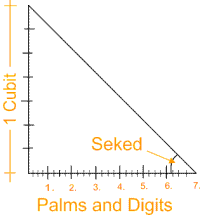Assessing the angle of slope of existing pyramids
The majority of all Egyptian pyramids are now in such a state of ruin that it is very difficult to assess the original height of the pyramid even if the base measurements can be accurately determined. In the few preserved pyramids there are a number of methods that can be used. The first involves measuring the slope of the remaining casing stones. It has been found that slight local variations occur. Averaging these give an approximate guide to the height of the pyramid. An example is the pyramid of Pepi II which I.E.S. Edwards estimated as being 171 feet and a base of 258 feet3. This gives an angle of slope of 52.97° determined from a few casing stones.

Flinders Petrie in his measurement of the Great Pyramid listed the angles of slope from the remaining casing stones as:

 Casing stones in situ on N. face = 51°-46'-45" Casing stones 5 overturned = 51°- 52' Casing stone fragments (18 no) = 51°-53'-4"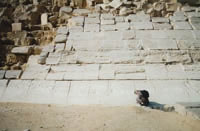Casing stones of the Northern Stone pyramid of Seneferu

To supplement these calculations Petrie carried out a number of additional checks involving accurately surveying the corner stone inclines and the height to the final existing level. This method has been adopted by other surveyors where applicable. None of the capstones, or pyramidions remain so completely accurate assessment is not possible. Nevertheless a very close approximation can be given particularly with the better preserved pyramids. Using all of these methods Petrie arrived at a figure of 51°-52' +/- 2' for the angle of slope of the Great Pyramid. Other pyramids on the Giza plateau had slightly greater variations depending upon their state of preservation. For example the pyramid of Menkaure is given as 51° +/- 10'.

Based on these methods the angles of slope of the best preserved pyramids from the IIIrd to VIth dynasties can be listed as follows:

 Maidum: N. Stone Pyramid: Bent pyramid(1): Bent pyramid(2): Khufu: Khafre: Menkaure (a): Menkaure (b): Userkaf: Sahure: Neferikare: Nyuserre: Teti: Pepi II: 51.88° 43.6° 54.52° 43.35° 51.87° 53.13° 51.19° 51.85° 53° 50.19° 53° 51.85° 53° 53°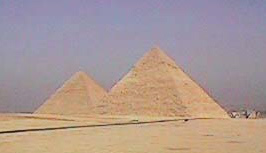The Pyramids of Khafre and Khufu are based on sekeds of 5.25 (5 palms and 1 digit) and 5.5 (5 palms and 2 digits) respectedly.

(Note: Recent evidence suggests that the pyramid of Menkaure was not set on a square base; one side being 335 feet whilst the other 343 feet. This will produce two different angles of slope one of 51.85° and the other of 51.19°.)

The angles of slope generated by the different sekeds can be shown as follows:

Seked      Degree Angle
4.00          60.25°
4.25          58.74°
4.50          57.26°
4.75          55.84°
5.00          54.46°
5.25          53.13°
5.50          51.84°
5.75          50.60°
6.00          49.40°
6.25          48.24°
6.50          47.12°
6.75          46.04°
7.00          45.00°
7.25          43.99°
7.50          43.02°

It has been assumed that only whole ratios would have been used in these calculations with the smallest unit being a digit. Practical building considerations would preclude the use of fractions of a digit although the answer to problem 56 RMP is given as a fraction.

From these tables it can be shown that the following sekeds were used more than once:

5.5       Maidum, Khufu, Nysuerre and Menkaure(b).
5.25     Khafre, Userkaf, Neferikare, Teti and Pepi II.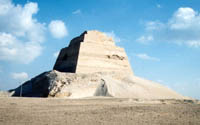The collapsed Pyramid of Maidum. Its angle of slope is the same as the Great Pyramid.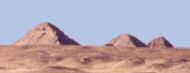The pyramids of Abusir - now in a bad state of repair, belonging to Sahure, Neferikare and Nysuerre.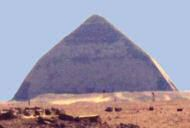The Bent Pyramid showing the change in angle of slope. Built by king Seneferu.

The pyramid of Sahure is probably based on a seked of 5.75 although its state of ruin makes accurate assessment difficult. The angle of slope of the lower portion of the Bent pyramid is likely based on a seked of 5. However the upper portion does not easily fit either a seked of 7.25 or 7.5. The same can also be applied to the Northern Stone pyramid and possibly also to the pyramid of Menkaure. This raises questions on whether the seked was the basis of the calculation in all cases. Before looking in more detail at this anomaly something needs to be said about the relevance of the known sekeds in the construction of the pyramids.

Pyramid design
It can be seen from the seked's used that two ratios were adopted on more than one occasion. These are the sekeds 5.5 [5 palms and 2 digits] and 5.25 [5 palms and 1 digit]. These two sekeds can be shown to be based on simple ratios; the first being a height to base of 7:11 and the second a height to base of 2:3 (4:6). This latter ratio is the derived from the 3 : 4 : 5 triangle.

We might question why the Ancient Egyptians would have wished to choose these sekeds when, for practical construction purposes, more simple seked ratios of 5 or 6 palms would have been more preferable. The first produces an angle of slope of 54.46° and the second of 49.4°. There is no technical constructional reasons why either of these angles could not have been adopted yet the only surviving pyramid that uses either of these ratios, is the lower portion of the Bent pyramid. We are therefore forced to ask what was so special about the sekeds of 5.5 and 5.25?

Seked 5.25 (ratio 3:4:5 triangle)
It has been claimed by a number of authorities that the Ancient Egyptians did not know the 3:4:5 Pythagorean ratio. For example T. L. Heath states in his book The Thirteen Books of Euclid's Elements, Vol 1:

"There seems to be no evidence that they (Egyptians) knew that triangle (3:4:5) is right-angled; indeed according to the latest authority (T. Eric Peet, The Rhind Mathematical Papyrus, 1923), nothing in Egyptian mathematics suggests that the Egyptians were acquainted with this or any special cases of the Pythagorean theorem."

This proposition is supported by Richard Gillings based on the known textual information. Set against this view there is considerable constructional and geometrical evidence to indicate that the Egyptians were well aware of the 3 : 4 : 5 ratio.

Firstly this triangle was used, or the ratios derived from it, in at least three pyramids including that of Khafre on the Giza plateau. Khafre's pyramid was measured by Petrie who gave the angle of slope as 53°-10' +/- 4'. We can therefore be fairly certain that the seked of 5.25, which produces an angle of 53°-7'-48", was intended in the construction.

It is clear from the cubit measures that have been recovered, that the Ancient Egyptians were quite capable of measuring to an accuracy of 1/16 of a digit. It is just not credible that they would never, whilst building or setting out their triangles, have bothered to measure the length of the hypotenuse of slope of the triangles based on their sekeds. Once a seked of 5.25 had been adopted, sooner or later someone would have measured the hypotenuse and discovered its relationship to the other two sides.

From other problems in the RMP it is clear that the Egyptians were quite capable of division. They would certainly have discovered that a triangle with sides of 6:8:10 (RMP 59) could be reduced to a 3:4:5 ratio. Indeed it could be argued that a whole number ratio of cubits, for the slope, base and height of the pyramid, was precisely why they chose to adopt a seked of 5.25. For this would considerably ease the technical problems of ensuring that the correct angle of slope was always maintained, in the final finishing of the casing stones. In support of this argument problems 57, 58 and 59 in the RMP are based on a seked of 5.25, which demonstrates its importance in pyramid design.

In addition there are two further items of corroborative evidence. The first stems from Pythagoras's exposure to Egyptian ideas during the ten years of his life that he spent in Egypt as part of the priesthood. Whilst it is very likely that he was the first individual to 'prove' the relationship between the sides of a right angled triangle, based on the square of its sides, we could also infer that he obtained a knowledge of the 3 : 4 : 5 triangle from his time in Egypt.

Secondly , whilst the problems in the RMP relate solely to the base and perpendicular sides of a right angled triangle we know that the hypotenuse was important in other calculations in relationship to areas. The unit of measure of a Remen, or more correctly double Remen is the diagonal of a square whose side is one cubit7. From this it is clear that the measure of the hypotenuse was an aspect of Ancient Egyptian mathematics and geometry which had practical application in the surveying of land.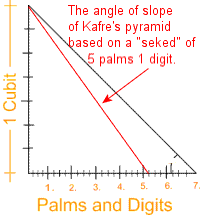Omitting all other evidence, that the seked of 5.25 (5 palms and 1 digit) occurs with such regularity both in the mathematic texts as well as in the practical construction of at least three pyramids lends substantial weight to the evidence that the 3 : 4 : 5 ratio was known to the Ancient Egyptians. Whether out of curiosity of intent the hypotenuse of a pyramid or triangle with this seked would have been accurately measured. This in turn would inevitably lead to a working knowledge of the 3 : 4 : 5 ratio.

Seked 5.5 (ratio 7:11 - height to base) - The Great Pyramid Ratio
We now need to question why a seked of 5.5 (5 palms and 2 digits) might have been used? This is not so obvious but an explanation could lie with a further understanding of Egyptian measures, particularly the relationship of the Royal cubit to the palm and the digit.

In modern times we are used to working with the metric system with its standard ten base ratios. Prior to this, in Britain, Imperial measures were used which incorporated a range of different ratios, 12 inches in one foot, 3 feet in one yard and so on. All ancient measures incorporate practical relationships to assist in the computation of lengths, areas and volumes. The relationship of the primary Ancient Egyptian measures has already been given. As a distance the cubit is generally reckoned to be 20.6 inches or 523 millimetres. For comparison the Sumerian cubit of 495 millimetres was divided into 30 digits as opposed to 28 digits in Egyptian measures. The Greeks also used a cubit of about 489 millimetres being divided into 24 digits. Both the Greeks and the Egyptians used 4 digits to equal a palm; giving 6 palms to one cubit in the case of Greek measures and 7 palms to one cubit in Egyptian measures.

In all ancient measures the division of the cubit into seven parts is, to say the least, very curious. As a measure it has no divisors being a prime number. It was probably for this reason that a short cubit was introduced of 6 palms, which could then be divided into halves and thirds; but not so the Royal cubit. It might be argued that the sevenfold division held some magical or numerological significance, such as a relationship to the 70 days between the rising and setting of the Dog Star, and that such a relationship has now been lost. There is however one practical reason why the Ancient Egyptians might have chosen to divide their cubit into seven parts.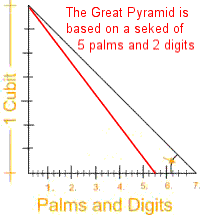Pi and the cubit
The most simple ratio for the pi (π) formula is 22/7, although today it is usually expressed decimally as 3.1416. Using the first of these two equations it becomes obvious that a circle with a radius of 7 units has a circumference of 44 units. In other words a circle with a radius of 1 cubit has a circumference of 44 palms. Using this method it is very easy to sub-divide the circumference into halves, quarters, eighths and sixteenths; there being 22 palms in a semi-circle, 11 palms in a quadrant and 5.5 palms in 1/8th of circle and 2.75 palms in 1/16th. In addition it is also very easy, through simple multiplication or division, to arrive at length of the circumference or a circle, or part thereof, once the radius is known. For example a radius of 2 cubits gives a circumference of 88 palms; 1.5 cubits has a circumference of 66 palms and so on.

There is no evidence in the mathematical texts that the Ancient Egyptians knew the 'pi' formula as such. In practice this would not be necessary for the formula was already contained within their measuring system through the simple adoption of the sevenfold division of the cubit.

Seen in this light it now becomes apparent why the Egyptians might have adopted a seked of 5.5 [5 palms and 2 digits] for the Great Pyramid and at least two other pyramids. As has often been stated the distance around the base of the Great Pyramid exactly equals the circumference of a circle whose radius is the height of the pyramid. Through the ratio of 7:11 there is a direct relationship between the three main elements in architecture, the square, the triangle and the circle. In the case of the Great Pyramid the circle is unseen, which equates with how it is often perceived in mystical tradition representing infinity, or the element of the divine.

In further support of these arguments the cubit measures of the Great Pyramid are generally given as base sides being 440 cubits and the height as 280 cubits, which are directly related to the numeric ratios stated above. The two numbers 7 and 11 can also be found woven into other elements of the pyramid's design. For example the height of the King's Chamber is 11 cubits and there are 7 corbels to each side of the Grand Gallery. These elements may have been coincidental, being applied for practical or aesthetic reasons. It is also possible that they may have contained a symbolic function in relation to the numeric ratios contained within the pyramids design.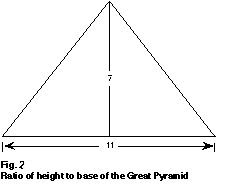Seked problems
Having considered reasons why seked's of 5.25 and 5.5 might have been used, there is still the problem of those pyramids which do not easily fit with the known seked ratios. These are the pyramids of Menkaure (51.19°), the Northern Stone Pyramid (43.6°) and the upper part of the Bent Pyramid (43.35°).

In examining these anomalies I first wish to focus on the Northern Stone Pyramid of Sneferu. Slight variations in the angle of slope are given by different authorities. For example I. E. S. Edwards gives an angle of 43°-36', whilst Mark Lehner in his book The Complete Pyramids suggests 43°-22' and Grinsell in his book "Egyptian Pyramids" gives 43°-40'. It should be remembered that accurate determination of the angle is difficult, although in the case of relatively intact pyramids, of which this is one, the best assessment can be made from measuring the length of the base and the size and height above base level of the highest remaining course. This gives reasonably accurate figures from which to calculate the angle of slope.

In a recent article by George Johnson, in KMT magazine (Vol. 8, no. 3), the angle of slope of the Northern Pyramid is given as 43°-36'-11", with an original base of 722 feet and a height of 343 feet. If these measurements are correct then the angle of slope can be shown to be 43° - 32' - 7". However the angle given suggests that the height measurement may not be completely accurate. To precisely fit an angle of 43° -36' -11" the height would need to be 343.812 feet.

This distances can be compared with the standard Egyptian measure of a Royal cubit. The base sides can be shown to be 420 cubits derived from a cubit length of 1.719 feet. Dividing this ratio into the height gives a figure of exactly 200 cubits (343.8 / 1.1719 = 200). The height to base ratio can therefore be shown to be 20:21 which conforms to the ratio figure given by Petrie. However this ratio does not fit a standard seked giving a measure of 7.35 sekeds. As already stated it, would not be practical, in constructional terms, to work with a seked based on a fraction of a digit.

All authorities suggest angles that fall around forty-three and a half degrees. This needs to be compared with sekeds of 7.25 which gives an angle of 43.99° and 7.5 which produces 43.02°. By way of comparison a pyramid with a base of 722 feet and a seked of 7.25 would have a height of 348.55 feet, whilst one with a seked of 7.5 would be 336.93 feet. Of these two measures 348.55 lies closest to the presumed height of 343.81 feet. However the discrepancy of 4.75 feet is greater than one would expect from an accurate survey. All of this evidence suggests that, on occasions, the Ancient Egyptians used different ratios for calculating their seked.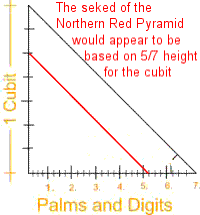This situation is further highlighted in the Bent Pyramid which also has an upper angle of around 43.5°. The angle of the lower half of this pyramid (54.52°) falls within 3.6' of a seked of 5.00 (54.46°), whilst the upper portion is nearly one third of a degree adrift from a seked of 7.5. If we assume that the measurements of this pyramid are accurate, which the seked for the lower portion suggests, then we are forced to the conclusion that variations for seked calculations must have occurred, despite the lack of textual evidence in RMP.

The ratio of 20:21 can be derived if the one cubit height is reduced by 5/7ths, which can be restated as working to 20 palms height rather than 28 for a full cubit.

The Pyramid of Menkaure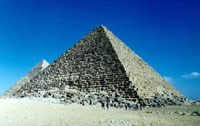The evidence from Menkaure's pyramid further supports this conclusion. As already stated Petrie gave the angle of slope of Menkaure's pyramid as
51° +/- 10'
whilst the figures from I. E. S. Edwards suggest an angle of 50°- 43'
The nearest equivalent seked to 51° is 5.75 which equals 50°- 36' or 5.5 which equals 51°- 51'.

Mark Lehner in his book The Complete Pyramids suggests that the base of Menkaure's pyramid is not square measuring 343 feet on one side and 335 feet on the other. If these figures are correct and the Egyptians built to a whole number of cubits then the longer side was probably based on 200 cubits and the shorter on 195 cubits. Applied to the pyramid this would give two angles of slope; one of 51.85° and the other of 51.19°. If these measurements are correct two different sekeds would have to have been used.

If the same idea, for the Northern Stone Pyramid of Sneferu, is applied to the pyramid of Menkaure, using a 5/7 seked ratio, then the angle of slope could be shown to be 4.00 sekeds which gives an angle of slope of 51.34° (51° - 20' - 25"). If this seked is correct then the height to base ratio is 5 to 8 for one of the angles of slope. Unfortunately this ratio does not easily fit to the other angle of slope which has a ratio of 7:11. This becomes apparent when the measures are converted to cubits.

As already states the longer side of 343 feet gives a length of almost exactly 200 cubits. This figure is divisible by 8 (200 / 8 = 25) and would give a theoretical height for the pyramid of 125 cubits. The shorter side would appear to be 195 cubits but in this case the number is not easily divisible by 11 (195 / 11 = 17.727), nor is the height of 125 cubits readily divisible by 7.

We might conclude that either the present measurements have not been determined with sufficient accuracy to draw conclusions or the builders failed to make the sides of this pyramid equal through faulty measurement. Because of the problems generated by two separate sekeds derived from a rectangular base it is very unlikely that an inaccurate ground plan could have gone un-noticed in the early stages of construction and not have been corrected. This suggests that, if the sides were not originally intended to be equal, then two separate sekeds were a deliberate aspect of the design. What these might have been cannot be easily determined.

Summary
Whilst the use of the seked, as indicated in RMP, can be clearly perceived in the design of a number of pyramids it cannot be conclusively shown to have been used in all. It is most likely that variations were used for specific reasons. With very few exceptions the ratios do not fit with basic building practice which would normally prefer to work with primary palm ratios such as 7 to 5. Instead we find the use of part palm ratios in almost all known pyramids. The only conclusion is that the symbolism of the ratio was more important than the ease of building practice. As has been demonstrated sekeds of 5.25 and 5.5 incorporate the 3 : 4 : 5 triangle and a relationship to circle respectively. It is therefore likely that it was these symbolic or cultic aspects that were deemed to be important in the design of the pyramids.

Notes:
1. Reading the Past: Mathematics and Measurement O.A.W. Dilke British Museum Press 1987
2. Mathematics in the Time of the Pharaohs Richard Gillings. Dover (NY) 1972
3. The Pyramids of Egypt I. E. S. Edwards. Ebury Press 1947
4. The Pyramids and Temples of Gizeh William Flinders Petrie. Field and Tuer 1883
5. The Pyramids Complete Mark Lehner. Thames and Hudson 1997
6. Egyptian Pyramids Leslie Grinsell John Bellows 1947

Sekeds and the Geometry of the Great Pyramid
Abydos - Temple mysteries
Egyptian Temple Orientation - Astronomical Alignments
Egypt tour : sixteen day tour in January 2008
The Keys to the Temple - Mystery patterns in the British landscape showing pyramid geometry.
Site Map - List of all David Furlong's articles

 For further information please write to: David Furlong Myrtles, Como Road, Malvern Worcs WR14 2TH or phone 01684-569105 or 07779789047                         Email: David FurlongDavid has been taking groups to Egypt for more than 15 years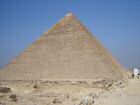The Great Pyramid of Giza built by King Khufu is based on an angle of slope of 5.5 sekeds.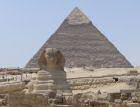The pyramid of King Kafre is based on an angle of slope of 5.25 seked.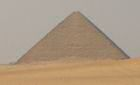The Red Pyramid of King Seneferu does not appear to be based on any whole number of sekeds, rather on a ratio of 20:21 (height:½base).

 All material copyright David Furlong 2010